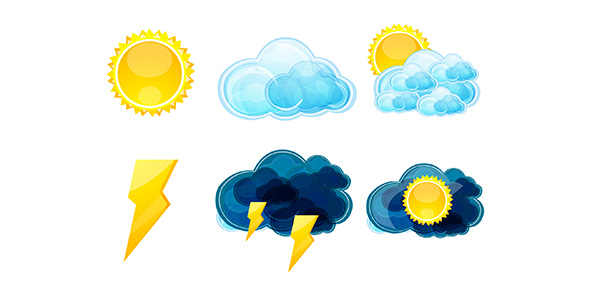# Weather Factors Part 2

20 Questions | Total Attempts: 184SettingsThis practice exam will help you study for the summative on Energy in teh Atmosphere and Heat Transfer

• 1.
Sunsets and sunrises are red because
• A.

Light from the sun is scattered leaving only red and blue light

• B.

Light from the sun is scattered leaving only red and orange light

• C.

The sun is higher in the sky

• D.

Heat in the atmosphere diffuses the light leaving red and orange light.

• 2.
Most of the energy from the sun travels to Earth in the form of
• A.

• B.

• C.

Visible light

• D.

Convection waves

• 3.
The direct transfer of heat from one substance to another through contact is called
• A.

• B.

Convection

• C.

Conduction

• D.

• 4.
The difference between temperature and thermal energy is
• A.

Temperature deals with the number of particles and thermal energy deals with heat output.

• B.

Temperature deals with heat output and thermal energy deals with the number of particles.

• C.

Temperature goes from higher to lower concentration while thermal energy goes from lower to higher concentration.

• D.

Temperature goes from lower to higher concentration while thermal energy goes from higher to lower concentration.

• 5.
In which state of matter are the molecules moving at a faster speed?
• A.

Solid

• B.

Liquid

• C.

Gas

• D.

They all move at the same speed

• 6.
The Earth’s surface is heated mainly by
• A.

• B.

Convection

• C.

Conduction

• D.

Evaporation

• 7.
Which of the following colors has the longer wavelength?
• A.

Red

• B.

Orange

• C.

Green

• D.

Blue

• 8.
The Celsius temperature scale is used
• A.

Only in America

• B.

By scientists to describe temperature

• C.

By weathermen to describe weather reports

• D.

Very rarely

• 9.
The formula for temperature conversion is  oC = 5/9 (oF – 32).  50 oF is converted to which of the following Celsius temperatures.
• A.

10 degrees Celsius

• B.

20 degrees Celsius

• C.

30 degrees Celsius

• D.

40 degrees Celsius

• 10.
Which of the following colors has a higher frequency
• A.

Red

• B.

Orange

• C.

Green

• D.

Blue

• 11.
Electromagnetic waves are classified by
• A.

Color

• B.

Speed

• C.

Wavelength

• D.

Visibility

• 12.
Energy from the sun travels to the Earth’s surface by
• A.

Evaporation

• B.

Conduction

• C.

Convection

• D.

Electromagnetic Waves

• 13.
The transfer of heat by the movement of fluid or gas is
• A.

• B.

Conduction

• C.

Convection

• D.

Evaporation

• 14.
Rising warm air carries thermal energy by
• A.

Conduction

• B.

• C.

Convection

• D.

Evaporation

• 15.
Air temperature is usually measured with a (n)
• A.

Thermometer

• B.

Aneroid Barometer

• C.

Mercury Barometer

• D.

Psychrometer

• 16.
Radiation, conduction and convection heat which layer of the Earth’s atmosphere?
• A.

Stratosphere

• B.

Mesosphere

• C.

Ionosphere

• D.

Thermosphere

• 17.
What percentage of the Sun’s energy is absorbed by the Earth’s surface (land and water)?
• A.

5%

• B.

20%

• C.

25%

• D.

50%

• 18.
What percentage of the Sun’s energy is reflected back into the Earth’s atmosphere by the surface of the Earth?
• A.

5%

• B.

20%

• C.

25%

• D.

50%

• 19.
What percentage of the Sun’s energy is reflected by clouds, dust and gasses?
• A.

5%

• B.

20%

• C.

25%

• D.

50%

• 20.
Infrared radiation is felt in the form of
• A.

Heat

• B.

Light

• C.

Sound

• D.

Convection waves

Related TopicsBack to top